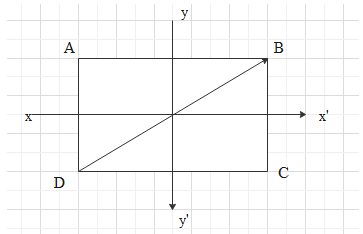In rectangle Axes and pass through the centre of the rectangle. The moment of inertia is least about

# In rectangleAxesandpass through the centre of the rectangle. The moment of inertia is least about

1. A
DB
2. B
BC
3. C4. DFill Out the Form for Expert Academic Guidance!l

+91

Live ClassesBooksTest SeriesSelf Learning

Verify OTP Code (required)

### Solution:

Concept: In this question we need to find out the axis through which moment of inertia is least.
Moment of inertia of a body about any axis is defined to be the product of masses and the distance of masses from its axis. Moment of inertia physically means the resistance that the body faces while rotating.
Considering the figure:And evaluating the options,
First of all, among DB and BC, BC will be rejected because it is not passing through the center of mass and it will not have the minimum moment of inertia.
Now by comparing DB,andwe see that DB does not have mass uniformly distributed along the axis, so it will not have the minimum moment of inertia. Hence, this is also rejected.
Fromand,will have the least moment of inertia because the mass is closely located from the axis ofso the answer isoption (3)
Hence, option 3 is correct.

## Related content

 Area of Square Area of Isosceles Triangle Pythagoras Theorem Triangle Formula Perimeter of Triangle Formula Area Formulae Volume of Cone Formula Matrices and Determinants_mathematics Critical Points Solved Examples Type of relations_mathematics+91

Live ClassesBooksTest SeriesSelf Learning

Verify OTP Code (required)